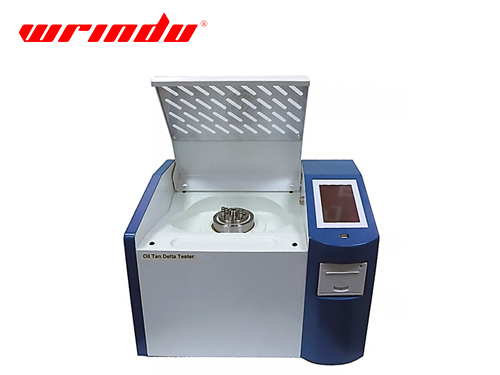# How to Measure the Dielectric Loss of Circuit-breaker Capacitor at High Voltage

24-02-2023

How to Measure the Dielectric Loss of  Circuit-breaker Capacitor at High VoltageINTRODUCTION

During the process of capacitance and dielectric loss measurement, due to coupling capacitance between test object and surrounding live parts, the measurement is disturbed by electric field so that real capacitance and dielectric loss cannot be obtained. In addition, according to related test standard, the test should use 10kV test voltage. However, with the improvement of voltage level, the dielectric loss data at 10kV always fails to reflect the conditions of equipment during the operation. To address the above-mentioned problem, the paper proposes that methods of using different frequency power source and boosting test voltage be utilized to measure the capacitance and dielectric loss.

1. Analysis of Improvements in Measuring Method

There are two problems in the on-site circuit-breaker capacitor test: ① Surrounding live components generate the electric field interference for test object so real capacitance and dielectric loss are not measured. ② The dielectric loss is so big at 10kV that the insulation condition cannot be reflected correctly during the running of capacitor.

Thus, this paper is to study the methods of improving circuit-breaker capacitance and dielectric loss test from two aspects: ① Methods to eliminate the interference of electric field. Use the power source which is different from power frequency to apply the voltage to test object and eliminate the interference component from signals. ② To study how the dielectric loss of capacitor varies according to the test voltage and boost the test power voltage to measure.

2. Test Scheme Brief

This paper proposes that methods of using different frequency power source and boosting test voltage be utilized to measure the capacitance and dielectric loss. Previously, only in the lab the dielectric loss could be measured at high voltage. As the test equipment downsizes, measurement of HV dielectric loss expands from lab to on-site.

3. On-site Test Results and Analysis

The above test scheme was applied in many substations. Test results were consistent with theoretical analysis. Take some 500kV circuit-breaker capacitor at Liu-dong substation as an example to introduce test process andresult analysis. The boosting mode of test transformer is used in the test. The test power source rises from 10kV to 80kV; measure one time every 10kV and then declines to 10kV; then measure one time every 10kV. As for each measuring voltage, perform capacitance and dielectric loss measurement at 49Hz and 51Hz. Its average value is taken as measuring value.

4. Conclusions

1) Through theoretical analysis, this paper proposes new test methods: use different frequency power source and digital filter to eliminate the interference from on-site live parts to test object and boost the test voltage. 2) Two dielectric loss measuring schemes are introduced: test transformer and series resonance. Their applicable ranges are compared: when the capacitance of test object ≤5000pF, test transformer or parallel reactor is recommended; when the capacitance ＞5000pF, the method of series resonance should be used. 3) Based on the above improvement methods and test schemes for dielectric loss measurement, tests are conducted on site at the substation. Test results indicate that new test methods can not only shield the electric field interference effectively, but also better reflects the insulation condition of capacitor during the running by means of measuring the dielectric loss.

Get the latest price? We'll respond as soon as possible(within 12 hours)